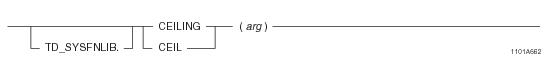# 15.00 - CEILING - Teradata Database

## Teradata Database SQL Functions, Operators, Expressions, and Predicates

Product
Release Number
15.00
Content Type
Programming Reference
Publication ID
B035-1145-015K
Language
English (United States)

## CEILING

### Purpose

Returns the smallest integer value that is not less than the input argument.

### Syntaxwhere:

 Syntax element… Specifies… TD_SYSFNLIB the name of the database where the function is located. arg a numeric expression.

### ANSI Compliance

This is a Teradata extension to the ANSI SQL:2011 standard.

### Invocation

CEILING is an embedded services system function. For information on activating and invoking embedded services functions, see “Embedded Services System Functions” on page 24.

### Argument Types and Rules

Expressions passed to this function must have one of the following data types:

BYTEINT, SMALLINT, INTEGER, BIGINT, FLOAT/REAL/DOUBLE PRECISION, DECIMAL/NUMERIC, or NUMBER

### Return Value

CEILING is a scalar function that returns the following values:

 IF arg is... THEN CEILING returns... a non-exact number the next integer value that is greater than arg. an exact number the input argument arg. NULL NULL.

### Result Type and Attributes

The result type is the same data type as that of the numeric input argument.

If the input argument is defined as a DECIMAL/NUMERIC with a precision less than 38, the return DECIMAL/NUMERIC value will have its precision increased by 1. For example, if DECIMAL(6,4) is passed in, it will be increased and returned as a DECIMAL(7,4). If the precision is 38, the scale will be reduced by 1 unless the scale is 0. For example, a DECIMAL(38,38) results in a return data type of DECIMAL(38,37).

For information on default data type formats, see SQL Data Types and Literals.

### Example

The following query returns the FLOAT value 16E0, since 16 is the smallest integer that is not less than the FLOAT value 15.7E0.

`   SELECT CEILING(157E-1);`

### Example

The following query returns a DECIMAL value of 16.0 since 16 is the smallest integer that is not less than the DECIMAL literal 15.7.

`   SELECT CEILING(15.7);`

### Example

The following query returns a DECIMAL value of -12.0 since -12 is the smallest integer that is not less than the DECIMAL literal -12.3.

`   SELECT CEILING(-12.3);`

### Example

The following query returns the value 10.00 with a data type of DECIMAL(4,2), since 10 is the smallest integer that is not less than 9.99. Note that the precision of the return value is increased by 1.

`   SELECT CEIL( CAST(9.99 AS DECIMAL(3,2)) );`